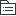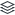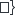# 14金融工具

2019年CPA考试会计第14章金融工具

98
|
29

## 相关模板推荐

•## 14金融工具 —— 作品大纲

•含义
• 形成一方的金融资产并形成其他方的金融负债或者权益工具的合同
•合同一方
• 金融资产
• 初始计量
• 公允价值
• 交易费用
• 以公允价值计量且变动计入当期损益的
• 交易费用进当期损益
• 除上面以外的
• 交易费用，倒挤在初始确认金额当中
• 发行、购买金融工具必须要花的钱
• 交易价值
• 一般为公允价值
• 有差额
• 通过透明合理的估值确定的，差额确认为利得或损失
• 其他方式确定的，差额递延
• 分类依据
• 业务模式与合同现金流量特征
• 业务模式
• 评估考虑因素
• 资产组合层次
• 关键管理人员决定的特定业务目标
• 以客观事实为依据
• 预期与现实有偏差
• 合同现金流量特征
• 反映经济特征的现金流量属性
• 类别
• 以摊余成本计量的金融资产
• 收息收本
• 会计科目
• 银行存款、贷款、应收账款、债权投资
• 后续计量
• 实际利率法
• 利息收入
• 基数
• 当期是否有信用损失
• =摊余成本=账面余额-减值准备
• =账面余额
• 特殊情况
• 其他债券投资，公允价值波动不影响摊余成本
• 利率
• 初始确认时是否有信用损失
• =经信用调整的实际利率
• =（账面余额-减值准备）在整个存续期的折现率
• =实际利率
• =账面余额在整个存续期的折现率
• 账务处理
• 初始确认
• 原则
• 初始金额=公允价值+交易费用
• 债权投资--成本
• 面值
• 应收利息/债权投资--应计利息
• 已宣告但未发放的应收利息
• 银行存款
• 实际花的钱，含交易费用
• 债权投资--利息调整
• 倒挤，可借可贷
• 后续计量
• 原则
• 实际利率法，摊余成本&实际利率
• 分期付息，到期还本
• 确认利息时
• 应收利息
• =双面=面值*票面利率
• 债权投资--利息调整
• 倒挤，与购入时相反
• 投资收益
• =摊余成本*实际利率
• 分次付息时
• 银行存款
• 应收利息
• 到期还本时
• 银行存款
• 债券投资--成本
• 面值
• 到期一次还本付息
• 确认利息时
• 债权投资--应计利息
• =双面=面值*票面利率
• 债权投资--利息调整
• 倒挤，与购入时相反
• 投资收益
• =摊余成本*实际利率
• 到期还本付息时
• 银行存款
• 债券投资--成本
• 面值
• 债权投资--应计利息
• 每期利息*期数
• 中途处置
• 原则
• 出售所得价款与账面价值差额进当期损益-投资收益/利息调整
• 出售导致的差额，调整摊余成本
• 债权投资--利息调整
• 投资损益
• 采用实际收到的钱和剩余部分的折现比较，差额部分计入此部分
• 银行存款
• 到手价，扣除交易费用
• 债权投资损失准备
• 如有
• 债权投资--成本
• 面值
• 债权投资--利息调整
• 未抵扣完的，最后一期倒着算
• 债权投资--应收利息
• 双面
• 投资损益
• 倒挤差额，可借可贷
• 以公允价值计量且其变动计入其他综合收益的金融资产
• 两者兼而有之：收本收息和出售获得短平快投资
• 综（债）
• 会计科目
• 其他债权投资
• 后续计量
• 初始确认
• 原则
• 初始费用=公允价值+交易费用
• 其他债权投资--成本
• 面值
• 应收利息/其他债权投资--应计利息
• 已宣告但未发放的应收利息
• 银行存款
• 实际花的钱
• 其他债权投资--利息调整
• 倒挤，可借可贷
• 后续计量
• 原则
• 公允价值变动计入其他综合收益
• 变动额为与摊余成本的差，但不影响摊余成本
• 升值
• 其他债权投资--公允价值变动
• 其他综合收益
• 升值的额度
• 贬值则反向
• 后续始终与摊余成本比较，扣减已经变动的额
• 股利/利息计提与发放
• 应收利息/其他债权投资--应计利息
• =双面=面值*票面利率
• 其他债权投资--利息调整
• 倒挤
• 投资收益
• 按摊余成本方式计算=账面余额*实际利率
• 处置时
• 原则
• 出售所得价款与账面价值差额进当期损益-投资收益/利息调整
• 将累计的其他综合收益结转入当期损益
• 处置时的投资收益
• 银行存款
• 到手价，扣除交易费用
• 其他债权投资--成本
• 面值
• 其他债权投资--应计利息
• 双面
• 其他债权投资--利息调整
• 倒着算，最初减去累计，可借可贷
• 其他债权投资--公允价值变动
• 累计的反向结转，可借可贷
• 投资损益
• 倒挤差额，可借可贷
• 结转累计其他综合收益
• 其他综合收益
• 累计的，可借可贷
• 投资损益
• 综（股）
• 将非交易性权益工具投资指定为综（股）
• 会计科目
• 其他权益工具投资
• 后续计量
• 初始确认
• 原则
• 初始费用=公允价值+交易费用
• 其他权益工具投资--成本
• 公允价值+交易费用
• 应收股利
• 已宣告但未发放的应收利息
• 银行存款
• 实际花的钱
• 持有期间
• 原则
• 公允价值变动计入其他综合收益
• 第一次与初始比，后面每次都与上期对比
• 股利收入
• 确认股利
• 应收股利
• =双面=面值*票面利率
• 投资收益
• 发放股利
• 银行存款
• 应收股利
• 公允价值变动
• 其他权益工具投资--公允价值变动
• 其他综合收益
• 或反向
• 不需计提减值准
• 终止确认
• 原则
• 出售所得价款与账面价值差额进留存收益
• 将累计的其他综合收益结转入留存收益
• 将投资卖出
• 银行存款
• 到手价，扣除交易费用
• 其他权益工具投资--成本
• 初始确认额
• 其他权益工具投资--公允价值变动
• 持有期间的累计，可借可贷
• 盈余公积
• 倒挤，可借可贷，一般根据留存收益的10%
• 利润分配--未分配利润
• 倒挤，可借可贷
• 其他综合收益的累计额转入留存收益
• 其他综合收益
• 公允价值变动造成的
• 累计的，可借可贷
• 盈余公积
• 利润分配--未分配利润
• 倒挤，可借可贷
• 以公允价值计量且其变动计入当期损益的金融资产
• 其他---托底
• 损（债）
•会计科目
• 交易性金融资产
• 损（股）
• 后续计量
• 初始确认
• 原则
• 交易费用计入当期损益
• 交易性金融资产--成本
• 倒挤
• 应收利息/应收股利
• 已宣告但未发放的应收利息
• 投资收益
• 交易费用
• 银行存款
• 实际花的钱
• 后续计量
• 原则
• 公允价值变动计入当期损益
• 第一次与初始比，后面每次都与上期对比
• 升值
• 交易性金融资产--公允价值变动
• 公允价值变动损益
• 升值的额度
• 贬值则反向
• 股利/利息计提与发放
• 宣告利息时
• 应收股利/应收利息
• =双面=面值*票面利率
• 投资收益
• 付息时
• 银行存款
• 应收股利/应收利息
• 处置时
• 原则
• 出售所得价款与账面价值差额进当期损益-投资收益/利息调整
• 将累计的公允价值变动损益计入当期损益
• 处置时的投资收益
• 银行存款
• 到手价，扣除交易费用
• 公允价值变动损益
• 升值的额度
• 交易性金融资产--成本
• 初始确认的反向结转
• 交易性金融资产--公允价值变动
• 累计的反向结转，可借可贷
• 投资损益
• 倒挤差额，可借可贷
• 结转累计公变损益
• 公允价值变动损益
• 累计的，可借可贷
• 投资损益
•合同其他方
• 金融负债
• 定义
• 交付资产、潜在不利的合同义务
• 自身权益工具结算的
• 非衍生工具，金融固定，数量可变
• 衍生工具，除固定数量换固定金额
• 特点：金额固定，必须还
• 初始计量
• 公允价值
• 同金融资产
• 分类
• 以公允价值计量且其变动计入当期损益的金融负债
• 类别
• 交易性金融负债
• 短平快+衍生
• 持有目的是近期出售或回购
• 初始确认是组合的一部分，但可短期获利
• 属于衍生工具（除财务担保合同和套期工具）
• 指定的金融负债
• 能有效消除会计错配
• 正式文件规定按公允价值进行管理评价
• 一经指定，不得撤销
• 非同控的或有对价
• 后续计量
• 公允价值，相关利得或损失计入当期损益
• 初始确认
• 原则
• 交易费用计入当期损益
• 银行存款
• 实际到手的钱
• 投资收益
• 交易费用
• 交易性金融负债--成本
• 持有期间
• 原则
• 公允价值变动计入当期损益
• 第一次与初始比，后面每次都与上期对比
• 计提与发放利息
• 宣告利息时
• 投资收益
• 应付利息
• =双面=面值*票面利率
• 付息时
• 应付利息
• =双面=面值*票面利率
• 银行存款
• 公允价值变动-减少
• 交易性金融负债--公允价值变动
• 公允价值变动损益
• 上升则反向
• 处置/偿还时
• 原则
• 偿还价款与账面价值差额进当期损益-投资收益/利息调整
• 将累计的公允价值变动损益计入当期损益
• 处置时的投资收益
• 交易性金融负债--成本
• 初始确认的反向结转
• 交易性金融负债--公允价值变动
• 累计的反向结转，可借可贷
• 银行存款
• 应偿还的本金+利息
• 投资损益
• 倒挤差额，可借可贷
• 结转累计公变损益
• 公允价值变动损益
• 累计的，可借可贷
• 投资损益
• 金融资产转移不符合终止确认条件或继续涉入被转移金融资产所形成的金融负债
• 后续计量
• 按金融资产转移相关计量
• 没指定的部分财务担保合同，以低于市场利率贷款的贷款承诺
• 后续计量
• 损失准备金额与初始确认减去累计摊销后的余额中的孰高计量
• 兜底，除上述以外的
• 后续计量
• 以摊余成本计量的金融负债
• 分类依据
• 自身业务特点和风险管理要求
• 权益工具
• 定义
• 除金融负债外的合同义务
• 自身权益工具结算的
• 非衍生工具，数量固定，除可变数量
• 衍生工具，固定数量换固定金额
• 特点：数量固定，不是必须还
• 会计处理
• 取得时，初始确认
• 银行存款
• 实际支付的金额
• 其他权益工具
• 持有期间，资产负债表日
• 不做处理
• 行权等终止确认时
• 固定金额换固定数量的股票
• 银行存款
• 换的金额-固定值
• 其他权益工具
• 初始确认的值
• 股本
• 固定数量乘以面值
• 资本公积--股本溢价
• 倒挤差额
• 两者区分
• 基本原则
• 是否必须还
• 金融负债
• 不能无条件赎回或强制付息
• 权益工具
• 是否数量固定
• 权益工具
• 固定数量
• 金融负债
• 数量可变，金额固定
• 需考虑因素
• 经济实质
• 工具特征
• 不需考虑因素
• 以前实施分配情况
• 未来实施分配情况
• 情形
• 固定金额的外币换固定数量的权益工具
• 考虑记账本位币，汇率变动，为金融负债
• 全部同类别的持有方按比例，为权益工具
• 或有结算条款
• 定义
• 发行方与持有方均不能控制
• 金融负债
• 发行方不能控制的因素，一旦发生就必须还
• 权益工具
• 特别罕见的情况下会发生
• 发行方清算时才需结算的
• 特殊金融工具中分类为权益工具的可回售工具
• 结算选择权
• 一般为金融负债/金融资产
• 合并报表中
• 站在集团的角度整体考虑
• 特殊金融工具的区分
• 可回售工具
• 一般金融负债
• 特殊权益工具
• 同时满足
• 清算时按比例获得净资产的权力
• 最次的级别
• 同类别的具有相同特征
• 不具有金融负债特征
• 按公允价值计算
• 总结
• 清算时还债后，具有按比例、同等分钱的权力，钱价为持有金融资产的公允价值
• 发行金融工具的重分类
• 权益工具→金融负债
• 公允价值
• 其他权益工具--优先股、永续债等
• 该工具的账面价值
• 应付债券--优先股、永续债等(利息调整)
• 该工具的公允价值减去应付债券的面值
• 应付债券--优先股、永续债等
• 面值
• 资本公积--股本溢价
• 该工具的公允价值减去权益工具的账面价值
• 不够冲减的依次冲减盈余公积
• 金融负债→权益工具
• 账面价值
• 应付债券--优先股、永续债等
• 面值
• 应付债券--优先股、永续债等(利息调整)
• 金融负债的账面价值减去面值
• 其他权益工具--优先股、永续债等
• 金融负债的账面价值
• 收益
• 金融负债
• 相关利息、股利、利得和损失进当期损益
• 权益工具
• 发行、回购、出售、注销时按权益变动处理
• 公允价值变动不做处理
• 分配做利润分配处理，不影响所有者权益总额
• 相关交易费用
• 新发行的直接相关费用，如为复合金融工具，按比例分摊
• 库存股
• 支付的对价和交易费用，确认较少所有者权益，不确认金融资产
• 库存股
• 银行存款
•分类
• 基础金融工具
• 债券，股票
• 衍生工具
• 期货，期权，互换
• 需同时满足
• 价值变动取决于标的变量的变化
• 不要求初始净投资
• 在未来某一日期结算
• 会计处理
• 取得时，初始确认
• 衍生工具
• 公允价值
• 投资收益
• 发生的交易费用
• 银行存款
• 实际支付的金额
• 持有期间，资产负债表日
• 公允价值高于账面余额的差额
• 衍生工具
• 公允价值变动损益
• 公允价值低于账面余额时则反向
• 卖掉等终止确认时
• 换现金
• 衍生工具
• 银行存款
• 换股票
• 衍生工具
• 股本
• 应付总价值除以市场价可兑换的股数
• 银行存款
• 股数不足整数的差价
• 资本公积--股本溢价
• 倒挤差额
• 复合金融工具
• 基本原则
• 非衍生金融工具及含有负债成分，又含有权益成分
• 可转换债券
• 初始分摊计算时
• 先确认负债成分（本金现值与利息年金现值）
• 实际到手的钱减负债获得权益成分
• 最后按比例分摊交易费用
• 转换时
• 终止确认负债成分，权益成分保留不变
• 会计处理
• 应付债券--可转换债券
• 用丁字帐计算
• 其他权益工具
• 初始确认时的账面
• 股本
• 面值
• 资本公积--股本溢价
• 倒挤差额
• 提前促成转换，所耗费的成本按比例分摊，转换获得的钱与公允价值之差确认为损失
• 到期没行权，将其他权益工具转为资本公积--股本溢价当中
•金融工具的计量和重分类
• 金融负债
• 金融工具的减值
• 金融工具的重分类
•金融资产转移
• 金融资产转移概述
• 金融资产转移的确认和计量
•套期会计
• 套期会计概述
• 套期工具和被套期项目
• 套期关系评估
• 确认与计量
• 信用风险敞口的公允价值选择权
•金融工具的披露
• 金融工具一般信息披露要求
• 资产负债表相关信息的披露
• 利润表相关信息的披露
• 套期会计相关披露
• 公允价值相关披露
• 金融工具风险信息相关披露
• 金融资产转移的披露